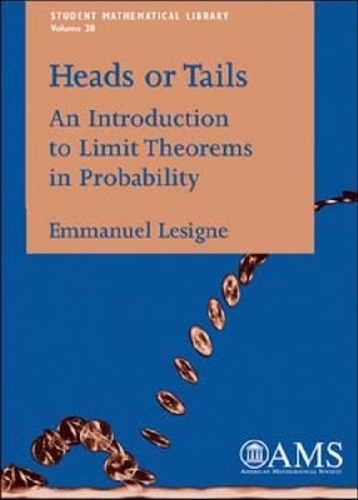•# Heads or Tails: An Introduction to Limit Theorems in Probability - Student Mathematical Library (Paperback)

(author)
£34.50
Paperback 150 Pages / Published: 30/06/2005
• We can order this

Usually dispatched within 15 working days

Everyone knows some of the basics of probability, perhaps enough to play cards. Beyond the introductory ideas, there are many wonderful results that are unfamiliar to the layman, but which are well within our grasp to understand and appreciate. Some of the most remarkable results in probability are those that are related to limit theorems - statements about what happens when the trial is repeated many times. The most famous of these is the Law of Large Numbers, which mathematicians, engineers, economists, and many others use every day.In this book, Lesigne has made these limit theorems accessible by stating everything in terms of a game of tossing of a coin: heads or tails. In this way, the analysis becomes much clearer, helping establish the reader's intuition about probability. Moreover, very little generality is lost, as many situations can be modelled from combinations of coin tosses. This book is suitable for anyone who would like to learn more about mathematical probability and has had a one-year undergraduate course in analysis.

Publisher: American Mathematical Society
ISBN: 9780821837146
Number of pages: 150
Weight: 202 g
Edition: illustrated Edition